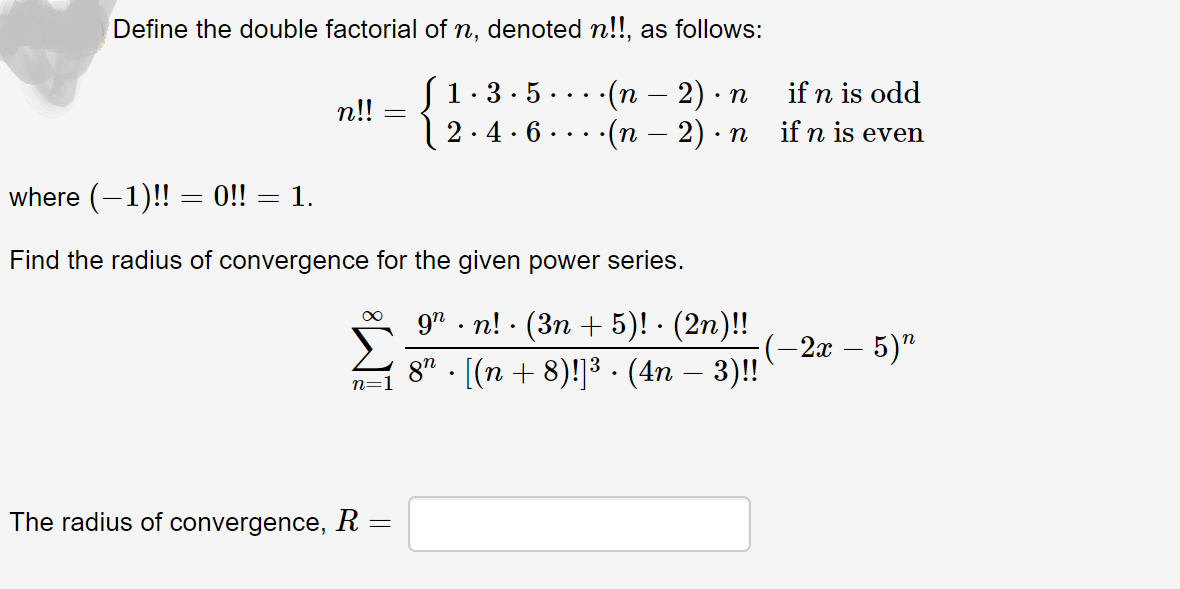# Define the double factorial of n, denoted n!!, as follows:S1.3.5| 2.4.6-(п — 2) п-(п — 2) 3if n is oddn!!if n is evenwhere (-1)! = 0!! = 1.%3DFind the radius of convergence for the given power series.9" . n! · (3n + 5)! - (2n)!!:(-2x – 5)"8" - [(n + 8)!]3 - (4n – 3)!The radius of convergence, R =

Question
37 viewshelp_outlineImage TranscriptioncloseDefine the double factorial of n, denoted n!!, as follows: S1.3.5 | 2.4.6 -(п — 2) п -(п — 2) 3 if n is odd n!! if n is even where (-1)! = 0!! = 1. %3D Find the radius of convergence for the given power series. 9" . n! · (3n + 5)! - (2n)!! :(-2x – 5)" 8" - [(n + 8)!]3 - (4n – 3)! The radius of convergence, R = fullscreen
check_circle

Step 1

The given power series is,

Step 2

Obtain the radius of convergence of the given power series as follows.

Step 3

By ratio test, the given powe...

### Want to see the full answer?

See Solution

#### Want to see this answer and more?

Solutions are written by subject experts who are available 24/7. Questions are typically answered within 1 hour.*

See Solution
*Response times may vary by subject and question.
Tagged in

### Other